Courses

# RD Sharma Solutions -Ex-20.4, Mensuration, Class 6, Maths Class 6 Notes | EduRev

## RD Sharma Solutions for Class 6 Mathematics

Created by: Abhishek Kapoor

## Class 6 : RD Sharma Solutions -Ex-20.4, Mensuration, Class 6, Maths Class 6 Notes | EduRev

The document RD Sharma Solutions -Ex-20.4, Mensuration, Class 6, Maths Class 6 Notes | EduRev is a part of the Class 6 Course RD Sharma Solutions for Class 6 Mathematics.
All you need of Class 6 at this link: Class 6

Question 1. Find the area of a rectangle, whose (i) Length = 6cm, breadth = 3 cm
(ii) Length = 8 cm, breadth = 3cm

(iii) Length = 4.5 cm, breadth = 2 cm
Solution: (i) Area of a rectangle = Length × Breadth
Length = 6 cm
Area of rectangle = 6 × 3 = 18 cm2
(ii) Area of a rectangle = Length × Breadth
Length = 8 cm Breadth = 3 cm
Area of rectangle = 8 × 3 = 24 cm2
(iii) Area of a rectangle = Length × Breadth
Length = 4.5 cm Breadth =2 cm
Area of rectangle = 4.5 × 2 = 9 cm2

Question 2. Find the area of a square whose side is: (i) 5 cm (ii) 4.1 cm (iii) 5.5 cm (iv) 2.6 cm
Answer: Area of a square = Side × Side (i) Side of the square = 5 cm
Area of the square = 5 × 5 = 25 cm2
(ii) Side of the square = 4.1 cm
Area of the square = 4.1 × 4.1 = 16.81 cm2
(iii) Side of the square = 5.5 cm
Area of the square = 5.5 × 5.5 = 30.25 cm2
(iv) Side of the square = 2.6 cm
Area of the square = 2.6 × 2.6 = 6.76 cm2

Question 3. The area of a rectangle is 49 cm2 and its breadth is 2.8 cm. Find the length of the rectangle.
Solution: Area = 49 cm2 Breadth = 2.8 cm
Area of the rectangle = Length × Breadth
Therefore, Length = Area / Breadth = 49 / 2.8 = 17.5 cm

Question 4. The side of a square is 70 cm. Find its area and perimeter.
Solution: Side of the square = 70 cm
Area of the square = Side × Side = 70 × 70 = 4900 cm2
Perimeter of the square = 4 × Side = 4 × 70 = 280 cm

Question 5. The area of a rectangle is 225 cm2 and its one side is 25 cm, find its other side.
Solution: Area = 225 cm2One of the sides = 25 cm Area of the rectangle = Product of the lengths of its two side Other side = Area / Side = 225 / 25 = 9 cm

Question 6. What will happen to the area of a rectangle if its
(i) Length and breadth are trebled
(ii) Length is doubled and breadth is same

(iii) Length is doubled
Solution: (i) If the length and breadth of a rectangle are trebled. Let the initial length and breadth be l and b, respectively.
Original area = l × b = lb
Now, the length and breadth are trebled which means they become three times of their original value.
Therefore New length = 3l
New breadth = 3b New area = 3l × 3b = 9lb
Thus, the area of the rectangle will become 9 times that of its original area.
(ii) If the length is doubled and the breadth is same. Let the initial length and breadth be l and b, respectively.
Original area = l × b = lb
Now, length is doubled and breadth remains same. Therefore New length = 2l
New area = 2l x b = 2 lb
Thus, the area of the rectangle will become 2 times that of its original area.
(iii) If the Length is doubled and breadth is halved.
Let the initial length and breadth be land b, respectively.
Original area =l × b = lb Now, length is doubled and breadth is halved.
Therefore New length = 2l
New breadth = b / 2
New area = 2 l × b / 2 = lb
New area is also lb. This means that the areas remain the same.

Question 7. What will happen to the area of a square if its side is:
(i) Tripled (ii) increased by half of it
Solution: (i) Let the original side of the square be s. Original area = s x s = s2
If the side of a square is tripled, new side will be equal to 3s.
New area = 3s × 3s = 9 s2 This means that the area becomes 9 times that of the original area.
(ii) Let the original side of the square be s. Original area = s x s = s2 If the side of a square is increased by half of it, new side =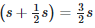New area =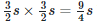This means that the area becomes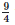times that of the original area.

Question 8. Find the perimeter of a rectangle whose area is 500 cm2 and breadth is 20 cm.
Solution: Area = 500 cm2 Breadth = 20 cm Area of rectangle = Length × Breadth
Therefore Length = Area / Breadth =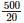= 25 cm
Perimeter of a rectangle = 2 (Length + Breadth) = 2 (25 + 20) cm = 2 × 45 cm = 90 cm

Question 9. A rectangle has the area equal to that of a square of side 80 cm. If the breadth of the rectangle is 20 cm, Find its length.
Solution: Side of the square = 80 cm Area of square = Side × Side = 80 x 80 = 6400 cm2
Given that: Area of the rectangle = Area of the square = 6400 cm2
Breadth of the rectangle = 20 cm
Applying the formula: Length of the rectangle = Area / Breadth We get: Length of the rectangle =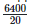= 320 cm

Question 10. Area of a rectangle of breadth 17 cm is 340 cm2 . Find the perimeter of the rectangle.
Solution: Area of the rectangle = 340 cm2Breadth of the rectangle = 17 cm
Applying the formula: Length of a rectangle = Area / Breadth We get: Length of the rectangle =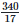=20 cm
Perimeter of rectangle = 2 (Length + Breadth) = 2 (20 + 17) = 2 x 37 = 74 cm

Question 11. A marble tile measures 15 cm × 20cm. How many tiles will be required to cover a wall of size 4m × 6m?
Solution: Dimensions of the tile = 15 cm × 20 cm
Dimensions of the wall = 4 m × 6 m = 400 cm × 600 cm (Since, 1 m = 100 cm)
Area of the tile = 15 cm × 20 cm = 300 cm2Area of the wall = 400 cm × 600 cm = 2,40,000 cm2
Number of tiles required to cover the wall =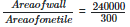= 800 tiles

Question 12. A marble tile measures 10 cm × 12 cm. How many tiles will be required to cover a wall of size 3m × 4m? Also, find the total cost of the tiles at the rate of Rs 2 per tile.
Solution: Dimension of the tile = 10 cm × 12 cm
Dimension of the wall =3 m × 4 m = 300 cm × 400 cm (Since, 1 m = 100 cm)
Area of the tile = 10 cm × 12 cm = 120 cm2 Area of the wall = 300 cm × 400 cm = 1,20,000 cm2
Number of tiles required to cover the wall = Area of wall / Area of one tile =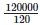= 1,000 tiles
Cost of tiles at the rate of Rs. 2 per tile = 2 x 1,000 = Rs. 2,000

Question 13. One tile of a square plot is 250 m, find the cost of leveling it at the rate of Rs 2 per square meter.
Solution: Side of the square plot = 250 m Area of the square plot = Side × Side = 250 × 250 = 62,500 m2
Rate of leveling the plot = Rs. 2 per m2 Cost of leveling the square plot = Rs. 62,500 × 2 = Rs. 1,25,000

Question 14. The following figures have been split into rectangles. Find the areas. (The measures are given in centimeters)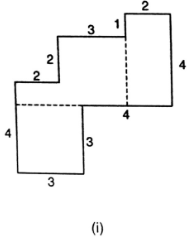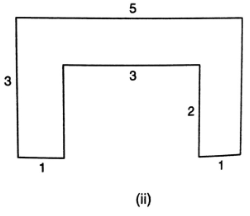Solution: (i)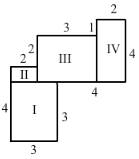This figure consists of two rectangles II and IV and two squares I and III.
Area of square I = Side × Side = 3 × 3 = 9 cm=2 Similarity, area of rectangle II = (2 × 1) = 2 cm2
Area of square III = (3 × 3) = 9 cm2 Area of rectangle IV = (2 x 4) = 8 cm2
Thus, the total area of this figure = (Area of square I + Area of rectangle II + Area of square III + Area of rectangle IV) = 9 + 2 + 9 + 8 = 28 cm2
(ii)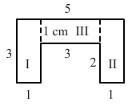This figure consists of three rectangles I, II and Ill.
Area of rectangle I = Length × Breadth = 3 × 1 = 3 cm2
Similarly, area of rectangle II = (3 × 1) = 3 cm2 Area of rectangle III = (3 × 1) = 3 cm2
Thus, the total area of this figure = (Area of rectangle I + area of rectangle II + area of rectangle III) = 3 + 3 + 3 = 9 cm2

Question 15. Split the following shapes into rectangles and find the area of each. (The measures are given in centimeters)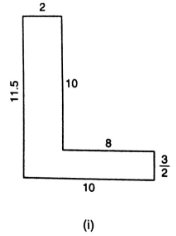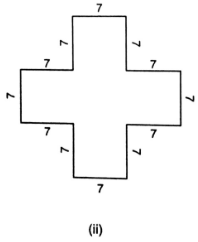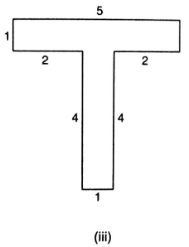Solution: (i) This figure consists of two rectangles I and II.
The area of rectangle I = Length × Breadth = 10 × 2 = 20 cm2
Similarly, area of rectangle II = 10 × 32 = 15 cm2 Thus, total area of this figure = (Area of rectangle I + Area of rectangle II) = 20 + 15= 35 cm2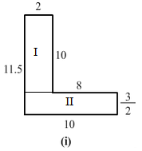(ii) This figure consists of two squares I and III and one rectangle II. Area of square I = Area of square III = Side x Side = 7 x 7 = 49 cm2
Similarly, area of rectangle II = (21 x 7) = 147 cm2
Thus, total area of this figure = (Area of square I + Area of rectangle II + Area of square III) = 49 + 49 + 147 = 245 cm2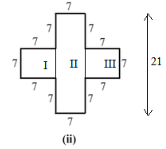(iii) This figure consists of two rectangles I and II. Area of rectangle I = Length x Breadth = 5 x 1 = 5 cm2
Similarly, area of rectangle II = 4 × 1 = 4 cm2
Thus, total area of this figure = (Area of rectangle I + Area of rectangle II) = 5 + 4 = 9 cm2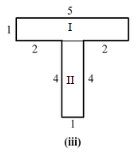Question 16. How many tiles with dimension 5 cm and 12 cm will be needed to fit a region whose length and breadth are respectively:
(i) 100 cm and 144 cm (ii) 70 cm and 36 cm
Solution: (i) Dimension of the tile = 5cm × 12 cm Dimension of the region = 100 cm × 144 cm
Area of the tile = 5 cm × 12 cm = 60 cm2 Area of the region = 100 cm × 144 cm = 14,400 cm2
Number of tiles required to cover the region = Area of the region / Area of one tile =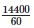= 240 tiles
(ii) Dimension of the tile = 5 cm × 12 cm Dimension of the region = 70 cm × 36 cm
Area of the tile = 5 cm × 12 cm = 60 cm2 Area of the region = 70 cm × 36 cm = 2,520 cm2
Number of tiles required to cover the region = Area of the region / Area of one tile =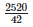= 42 tiles

103 docs

,

,

,

,

,

,

,

,

,

,

,

,

,

,

,

,

,

,

,

,

,

,

,

,

,

,

,

,

,

,

;